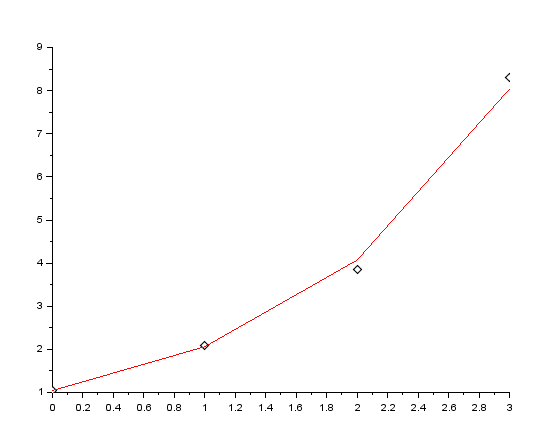# Exponential Fitting -SCILAB

I have written a code that will exponentially fit a given set of data-points.(Yfit=c*e^(a*x))

The procedure is based on least square approximation, which, in simple words,works by finding a line that is at a minimum distance possible from all the points.

Let’s say you have the x-axis points stored in a matrix, ‘x’ & the y-axis points stored in a matrix ‘y’. Then the following code returns the value of the coefficient, ‘c’ and ‘a’ such that the equation of the fitted line is y=c*e^(a*x), and also the fitted points which are basically the points of the y-axis obtained from the equation of the best fit-line.

CODE:

```//Exponential Fitting
//To exponentially fit a given set of data-points.
//Written By: Manas Sharma(www.bragitoff.com)
funcprot(0);
function [f,a,c]=expofit(x,y)
n=size(x);
if n(2)>n(1) then
n=n(2)
else
n=n(1);
end
for i=1:n
Yln(i)=log(y(i));
end
xsum=0;
ysum=0;
xysum=0;
x2sum=0;
for i=1:n
xsum=x(i)+xsum;
ysum=Yln(i)+ysum;
x2sum=x(i)*x(i)+x2sum;
xysum=x(i)*Yln(i)+xysum;
end
a=(n*xysum-xsum*ysum)/(n*x2sum-xsum*xsum);
b=(x2sum*ysum-xsum*xysum)/(x2sum*n-xsum*xsum);
c=exp(b);
for i=1:n
f(i)=c*exp(a*x(i));
end
endfunction

```

Sample Demo:

```x=[0,1,2,3];
y=[1.05,2.1,3.85,8.3];
[yfit,a,c]=expofit(x,y)

```

Output:

```c  =

1.0433996
a  =

0.6808532
yfit  =

1.0433996
2.0613012
4.0722295
8.044944
```

We can now even plot the observed data-points and the fitted points(yfit) and compare the two.

```plot2d(x,y,-5)
plot2d(x,yfit,5)
```I have created a module in SCILAB which contains the above macro, and once installed can be used as an in-built function. You can download it from here: https://atoms.scilab.org/toolboxes/curvefit

Leave your questions/suggestion/corrections in the comments section down below and I’ll get back to you soon.[wpedon id="7041" align="center"]

## One thought on “Exponential Fitting -SCILAB”

1. Great work. I shall appreciate if you can also cover exponential fit of the form y = a0 + (a1 * exp (x/b1)) + (a2 * exp (x/b2)) and share the code.